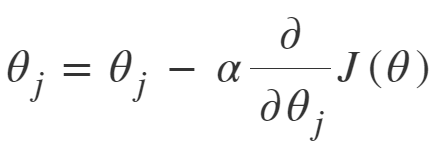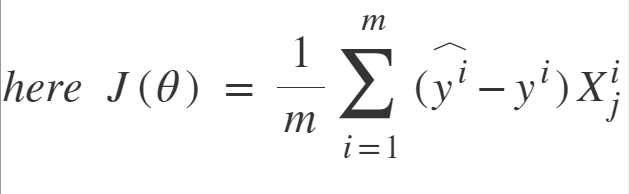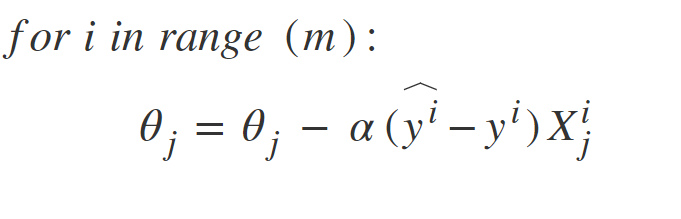# Difference between Batch Gradient Descent and Stochastic Gradient Descent

• Difficulty Level : Medium
• Last Updated : 15 Sep, 2022

In order to train a Linear Regression model, we have to learn some model parameters such as feature weights and bias terms. An approach to do the same is Gradient Descent which is an iterative optimization algorithm capable of tweaking the model parameters by minimizing the cost function over the train data. It is a complete algorithm i.e it is guaranteed to find the global minimum (optimal solution) given there is enough time and the learning rate is not very high. Two Important variants of Gradient Descent which are widely used in Linear Regression as well as Neural networks are Batch Gradient Descent and Stochastic Gradient Descent(SGD). Batch Gradient Descent: Batch Gradient Descent involves calculations over the full training set at each step as a result of which it is very slow on very large training data. Thus, it becomes very computationally expensive to do Batch GD. However, this is great for convex or relatively smooth error manifolds. Also, Batch GD scales well with the number of features.Stochastic Gradient Descent: SGD tries to solve the main problem in Batch Gradient descent which is the usage of whole training data to calculate gradients at each step. SGD is stochastic in nature i.e. it picks up a “random” instance of training data at each step and then computes the gradient, making it much faster as there is much fewer data to manipulate at a single time, unlike Batch GD.There is a downside of the Stochastic nature of SGD i.e. once it reaches close to the minimum value then it doesn’t settle down, instead bounces around which gives us a good value for model parameters but not optimal which can be solved by reducing the learning rate at each step which can reduce the bouncing and SGD might settle down at global minimum after some time.

## Difference between Batch Gradient Descent and Stochastic Gradient Descent

My Personal Notes arrow_drop_up
Recommended Articles
Page :